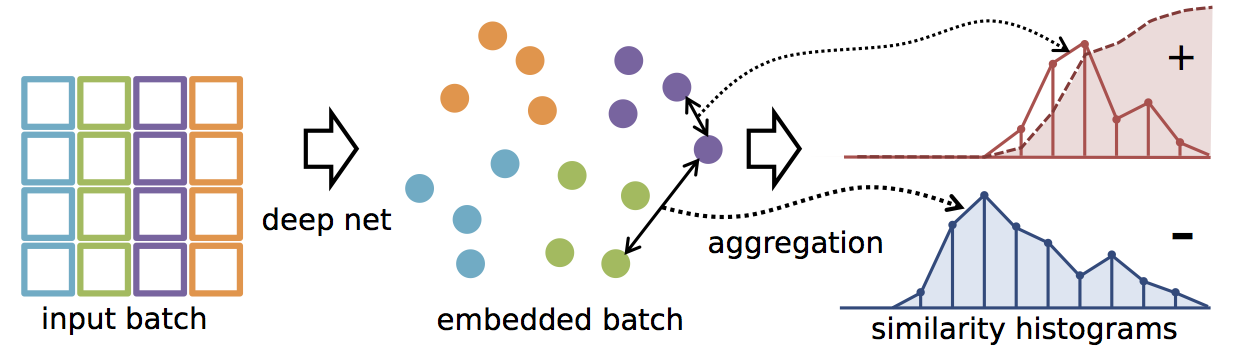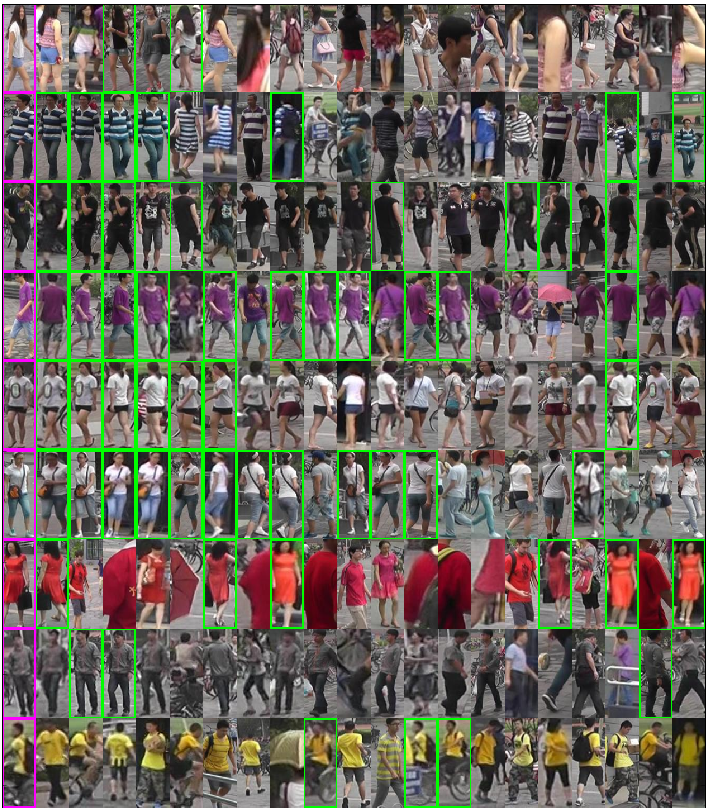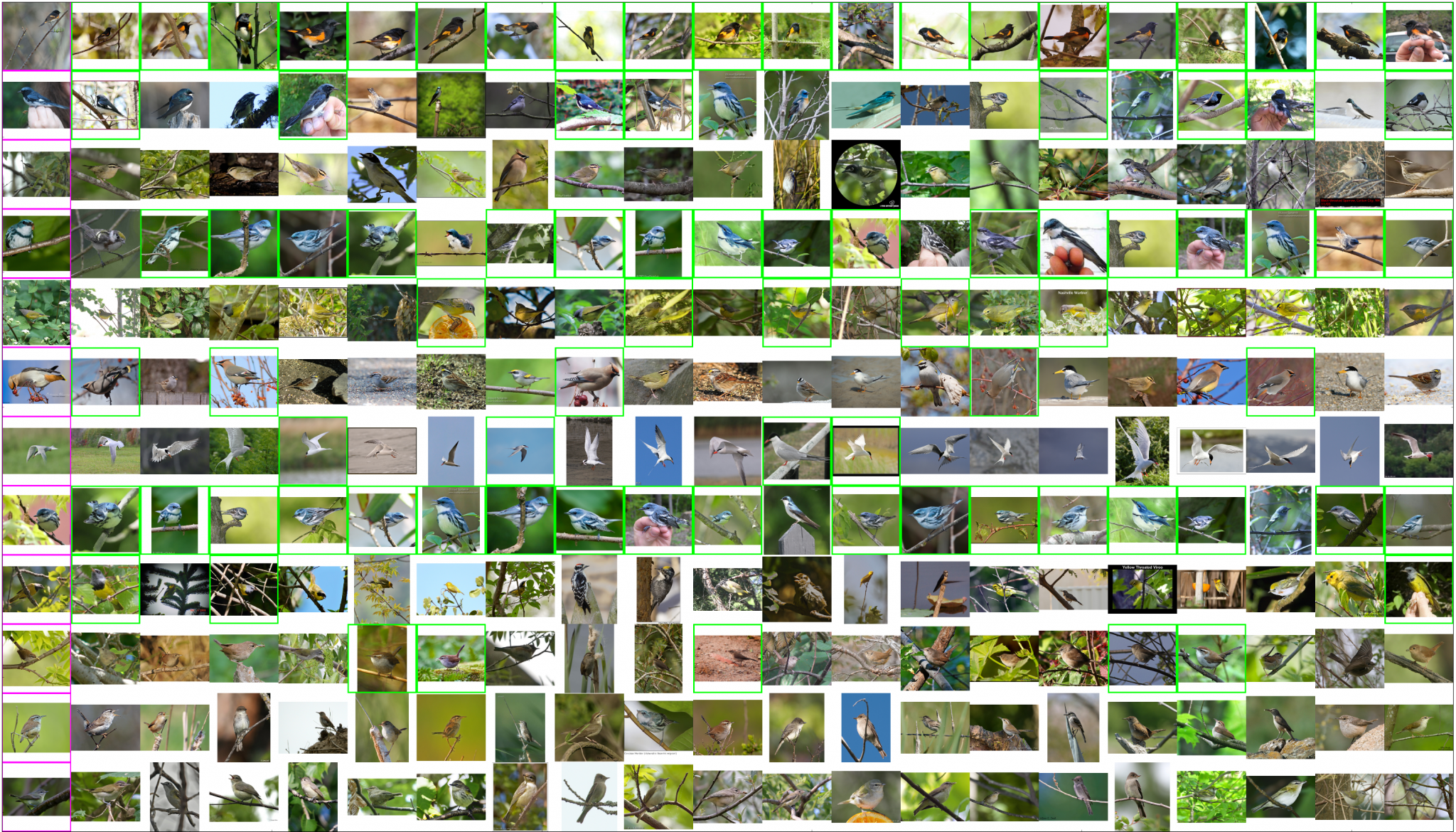# Learning Deep Embeddings With Histogram Loss

#### Evgeniya Ustinova, Victor Lempitsky

At NIPS2016 (paper),  github (caffe-code, pytorch-code),  poster (pdf)

# Abstract

We suggest a new loss for learning deep embeddings. The key characteristics of the new loss is the absence of tunable parameters and very good results obtained across a range of datasets and problems. The loss is computed by estimating two distribution of similarities for positive (matching) and negative (non-matching) point pairs, and then computing the probability of a positive pair to have a lower similarity score than a negative pair based on these probability estimates. We show that these operations can be performed in a simple and piecewise-differentiable manner using 1D histograms with soft assignment operations. This makes the proposed loss suitable for learning deep embeddings using stochastic optimization. The experiments reveal favourable results compared to recently proposed loss functions.

The learning objective is to achieve the proximity of semantically-related images and avoid the proximity of semantically-unrelated images.

# Approach

After the batch (left) is embedded into a high-dimensional space by a deep network (middle), we
compute the histograms of similarities of positive (top-right) and negative pairs (bottom-right). We then evaluate
the integral of the product between the negative distribution and the cumulative density function for the positive
distribution (shown with a dashed line), which corresponds to a probability that a randomly sampled positive
pair has smaller similarity than a randomly sampled negative pair. Such histogram loss can be minimized by
backpropagation. The only associated parameter of such loss is the number of histogram bins, to which the
results have very low sensitivity.# Results

Recall@K for (left) – CUHK03 and (right) – Market-1501 datasets. The Histogram loss outperforms Binomial Deviance, LSSS and Triplet losses :

Examples of output for random queries for Market-1501. First column contains query images,  the retrieved images are sorted by similarity in the descending order. Ground truth images are highlighted in green.Examples of output for random queries for CUB-200-2011: